# java while语句_Java while循环语句

Java while循环语句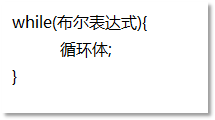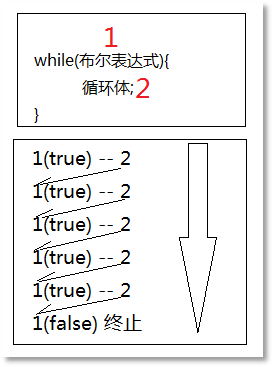while(true){

System.out.println("死循环");

}

int i = 0;

while(i < 10){

i++;

System.out.println("i = " + i);

}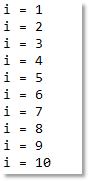int j = 1;

while(j <= 10){

System.out.println("j = " + j);

j++;

}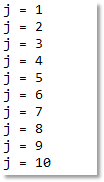int i = 0;

while(i < 10){

System.out.println("i = " + (++i));

}

int j = 1;

while(j <= 10){

System.out.println("j = " + j++);

}

int sum = 0;

int i = 0;

while(i <= 100){

if(i % 2 == 0){

sum += i;

}

i++;

}

System.out.println("sum = " + sum);

sum = 0;

int j = 0;

while(j <= 100){

sum += j;

j += 2;

}

System.out.println("sum = " + sum);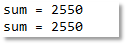08-01192
11-28312

01-176万+
11-105475
12-092964
05-232432
07-159352
06-2562
03-16522
07-193613
06-19267
06-024419
07-211万+
08-28152
03-121万+
02-161182
07-314316
04-061万+
09-021万+
06-301万+

### “相关推荐”对你有帮助么？

•非常没帮助
•没帮助
•一般
•有帮助
•非常有帮助¥2 ¥4 ¥6 ¥10 ¥20余额支付 (余额：-- )扫码支付获取中扫码支付点击重新获取扫码支付1.余额是钱包充值的虚拟货币，按照1:1的比例进行支付金额的抵扣。
2.余额无法直接购买下载，可以购买VIP、C币套餐、付费专栏及课程。余额充值